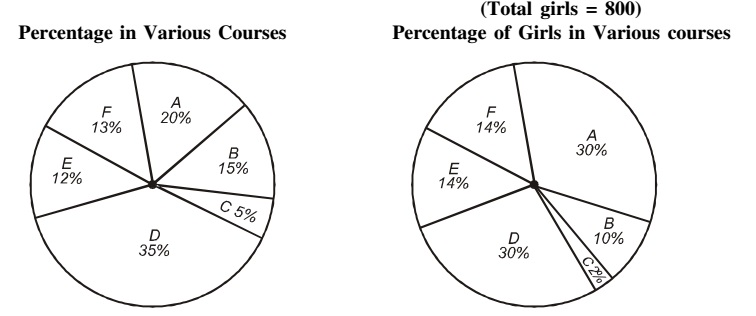## Pie chart

#### Data Interpretation

Direction: Study the following Graph carefully and answer the questions given below.
Percentage of students in various courses (A, B, C, D, E, F) and Percentage of girls out of these.
Total students = 1200 (800 girls + 400 boys)1. How many girls are there in course C?

1. As per second graph,
Number of girls in course 'C' = Total number of Girls x 2%

##### Correct Option: A

As per second graph,
Number of girls in course 'C' = Total number of Girls x 2%
Number of girls in course 'C' = 800 x 2/100 = 16

1. For which pair of courses is the number of boys the same?

1. As per first graph,
The number of students for course A = Total number of students x 20%
As per second graph,
The number of girls for course A = Total number of girls x 30%
Number of boys in course 'A' = The number of students for course A - The number of girls for course A

Same formula can apply for the other courses also.

##### Correct Option: A

As per first graph,
The number of students for course A = Total number of students x 20%
As per second graph,
The number of girls for course A = Total number of girls x 30%
Number of boys in course 'A' = The number of students for course A - The number of girls for course A
Number of boys in course 'A' = (1200 x 20/100 ) - ( 800 x 30/100 ) = 240 - 240 = 0
Similarly,
Number of boys in course 'B' = (1200 x 15/100 ) - (800 x 10/100) = 180 - 80 = 100
Number of boys in course 'C' = (1200 x 5/100 ) - (800 x 2/100) = 60 - 16 = 44
Number of boys in course 'D' = (1200 x 35/100 ) - (800 x 30/100) = 420 - 240 = 180
Number of boys in course 'E' = (1200 x 12/100 ) - 800 x 14/100 ) = 144 - 112 = 32
Number of boys in course 'F' = (1200 x 13/100 ) - ( 800 x 14/100 ) = 156 - 112 = 44
Hence, number of boys in course 'C' and 'F' are same which is 44.

1. For course E, the number of girls is how much percent more than the boys for course E?

1. For course E,
Number of boys = ( 1200 x 12/100 ) - (800 x 14/100 ) = 144 - 112 = 32

##### Correct Option: C

For course E,
Number of boys = ( 1200 x 12/100 ) - (800 x 14/100 ) = 144 - 112 = 32
Number of girls = 800 x 14/100 = 112
Required percentage = ( (112 - 32 )/32 ) x 100 = (80/32) x 100 = 250%

1. For which course is the number of boys the minimum?

1. As per first graph,
The number of students for course A = Total number of students x 20%
As per second graph,
The number of girls for course A = Total number of girls x 30%
Number of boys in course 'A' = The number of students for course A - The number of girls for course A

##### Correct Option: A

As per first graph,
The number of students for course A = Total number of students x 20%
As per second graph,
The number of girls for course A = Total number of girls x 30%
Number of boys in course 'A' = The number of students for course A - The number of girls for course A
Number of boys in course 'A' = (1200 x 20/100 ) - ( 800 x 30/100 ) = 240 - 240 = 0
Number of boys in course 'C' = (1200 x 5/100 ) - (800 x 2/100) = 60 - 16 = 44
Number of boys in course 'E' = (1200 x 12/100 ) - 800 x 14/100 ) = 144 - 112 = 32
Number of boys in course 'F' = (1200 x 13/100 ) - ( 800 x 14/100 ) = 156 - 112 = 44
Hence, in course 'A' number of boys is minimum which is Zero.

1. For course D what is the respective ratio of boys and girls?

1. As per first graph,
The number of students for course D = Total number of students x 20%
As per second graph,
The number of girls for course D = Total number of girls x 30%
Number of boys in course 'D' = The number of students for course D - The number of girls for course D

##### Correct Option: A

As per first graph,
The number of students for course D = Total number of students x 20%
As per second graph,
The number of girls for course D = Total number of girls x 30%
Number of boys in course D = The number of students for course D - The number of girls for course D
Number of boys = ( 1200 x 35/100 ) - ( 800 x 30/100 ) = 420 - 240 = 180
Number of girls = 800 x 30/100 = 240
Since, required ratio = 180 : 240 = 3 : 4